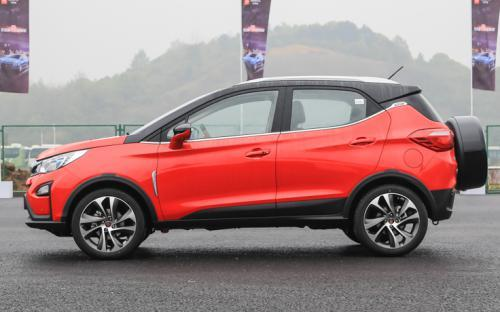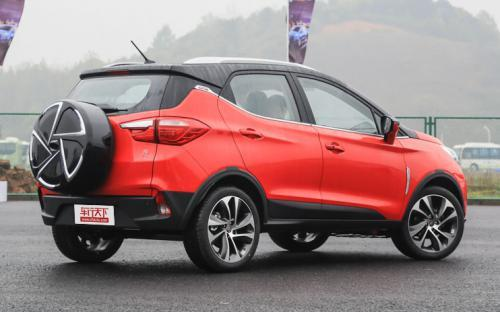### 比亚迪 元7 种颜色可选2016款最低售价：24.98 万元起

4360(mm)1785(mm)1650(mm)##### 配置亮点：
• 胎压监测装置

• ISOFIX儿童座椅接口

• 车身稳定控制(ESC/ESP/DSC等)

• 电动天窗

• 定速巡航

• 后倒车雷达

• 真皮座椅

• GPS导航系统

• 氙气大灯

• 后视镜加热

• 提交
2016款 1.5L 手动尊贵型 (129张)
• 2016款 1.5L 手动尊贵型 (129张)
• 2016款 1.5TID 自动旗舰型 (104张)
• 2016款 双模版 1.5L 全时四驱旗舰型 (111张)
• 2016款 1.5L 手动豪华型 (98张)
• 比亚迪 元 绕车实拍• 比亚迪 元 在售车型

排量 车型 厂商指导价 本地最低报价 购车工具
1.5L
5.99万
5.99万

6.59万
6.99万
7.59万
7.99万
8.59万
8.59万

双模版 1.5L 全时四驱豪华型 油电混合 6挡双离合
20.98万
双模版 1.5L 全时四驱尊贵型 油电混合 6挡双离合
22.98万
双模版 1.5L 全时四驱旗舰型 油电混合 6挡双离合
24.98万
24.98万

1.5T
1.5TID 自动标准型 6挡双离合
8.59万
1.5TID 自动舒适型 6挡双离合
8.99万
1.5TID 自动豪华型 6挡双离合
9.59万
1.5TID 自动尊荣型 6挡双离合
10.59万
1.5TID 自动旗舰型 6挡双离合
12.19万

### 比亚迪 元 动力加速

0-100公里加速时间分布在 4.9-4.9秒 属于 高性能级

动力级别 加速时间 车型
高性能级(1款)
4.9s
双模版 1.5L 全时四驱豪华型双模版 1.5L 全时四驱尊贵型双模版 1.5L 全时四驱旗舰型

比亚迪 元 视频

比亚迪 元 新闻资讯

# 长安CS15正式上市 售价5.79-7.39万元

上市新车 超过9028次关注

2016年4月6日，长安汽车正式宣布，全新SUV长安CS15正式上市，新车定位为一款入门级的小型SUV。全新小型SUV车型新车共会推出4款车型，搭载1.5L自然吸气发动机，售价...

# 比亚迪元正式上市 售价5.99-24.98万元

上市新车 超过8609次关注

2016年4月11日，比亚迪旗下全新小型SUV元正式上市。新车搭载1.5L、1.5T两款发动机，同时还推出插电混动版车型。新车共推出14款车型，售价区间为5.99-24.98万元，

# 2015上海车展 比亚迪新SUV元首发亮相

国产新车 超过8251次关注

上海国际车展上，比亚迪全新车型——比亚迪元首发亮相。作为一款小型新能源SUV，可以看作为亚迪S1的新能源版本。新车采用比亚迪家族设计，据悉，比亚迪元将在今年第...

# 盘点四月份即将上市的新车 总有一款适合您

即将上市 超过8335次关注

一转眼就到了4月份的第二周，本周将上市7款新车，自主、合资、进口均有，包括比亚迪元、国产XT5、全新思域、风神A9、AX7、蔚揽等。

# 三种动力配备选择 比亚迪元4月11日上市

国产新车 超过8481次关注

据官方获悉，比亚迪正式公布全新SUV比亚迪元，新车会推出燃油版以及插电式混合动力版本两种动力形式，共三款动力配备的车型。据悉，比亚迪元将在4月11日正式上市。

猜你喜欢

﻿
• 快速找车
• 选择品牌
• 选择品牌
• A  奥迪
• A  阿斯顿·马丁
• A  阿尔法·罗密欧
• B  宝沃
• B  布加迪
• B  巴博斯
• B  保时捷
• B  宾利
• B  奔驰
• B  宝马
• B  本田
• B  别克
• B  标致
• B  比亚迪
• B  宝骏
• B  北汽制造
• B  北汽新能源
• B  北汽幻速
• B  北汽威旺
• B  北京汽车
• B  奔腾
• B  北汽绅宝
• C  长安
• C  长安商用
• C  长城
• C  昌河
• D  大众
• D  道奇
• D  DS
• D  东南
• D  东风风神
• D  东风风行
• D  东风小康
• D  东风风度
• D  东风
• F  福特
• F  丰田
• F  菲亚特
• F  法拉利
• F  福田
• F  福迪
• F  福汽启腾
• G  观致
• G  广汽传祺
• G  广汽吉奥
• G  GMC
• H  红旗
• H  汉腾汽车
• H  哈弗
• H  哈飞
• H  海格
• H  海马
• H  华颂
• H  黄海
• H  华泰
• H  恒天
• J  吉利汽车
• J  捷豹
• J  Jeep
• J  江淮
• J  江铃
• J  金杯
• J  九龙
• J  金旅
• K  凯翼
• K  凯迪拉克
• K  克莱斯勒
• K  科尼塞克
• K  卡威
• K  开瑞
• L  路虎
• L  林肯
• L  劳斯莱斯
• L  兰博基尼
• L  雷克萨斯
• L  铃木
• L  雷诺
• L  理念
• L  力帆
• L  莲花汽车
• L  猎豹
• L  路特斯
• L  陆风
• M  马自达
• M  MG
• M  MINI
• M  玛莎拉蒂
• M  摩根
• M  迈凯轮
• N  纳智捷
• O  欧宝
• O  讴歌
• O  欧朗
• Q  奇瑞
• Q  起亚
• Q  启辰
• R  日产
• R  荣威
• R  瑞麒
• S  三菱
• S  斯威汽车
• S  萨博
• S  smart
• S  斯柯达
• S  斯巴鲁
• S  思铭
• S  双龙
• S  上汽大通
• S  双环
• T  特斯拉
• T  腾势
• W  沃尔沃
• W  五菱汽车
• W  五十铃
• W  威兹曼
• W  威麟
• X  现代
• X  雪佛兰
• X  雪铁龙
• X  西雅特
• Y  一汽
• Y  英菲尼迪
• Y  英致
• Y  依维柯
• Y  野马汽车
• Y  永源
• Z  众泰
• Z  中华
• Z  中兴
• Z  知豆
• 选择车系
• 选择车系
• 车型对比
• 选择品牌
• 选择品牌
• A  奥迪
• A  阿斯顿·马丁
• A  阿尔法·罗密欧
• B  宝沃
• B  布加迪
• B  巴博斯
• B  保时捷
• B  宾利
• B  奔驰
• B  宝马
• B  本田
• B  别克
• B  标致
• B  比亚迪
• B  宝骏
• B  北汽制造
• B  北汽新能源
• B  北汽幻速
• B  北汽威旺
• B  北京汽车
• B  奔腾
• B  北汽绅宝
• C  长安
• C  长安商用
• C  长城
• C  昌河
• D  大众
• D  道奇
• D  DS
• D  东南
• D  东风风神
• D  东风风行
• D  东风小康
• D  东风风度
• D  东风
• F  福特
• F  丰田
• F  菲亚特
• F  法拉利
• F  福田
• F  福迪
• F  福汽启腾
• G  观致
• G  广汽传祺
• G  广汽吉奥
• G  GMC
• H  红旗
• H  汉腾汽车
• H  哈弗
• H  哈飞
• H  海格
• H  海马
• H  华颂
• H  黄海
• H  华泰
• H  恒天
• J  吉利汽车
• J  捷豹
• J  Jeep
• J  江淮
• J  江铃
• J  金杯
• J  九龙
• J  金旅
• K  凯翼
• K  凯迪拉克
• K  克莱斯勒
• K  科尼塞克
• K  卡威
• K  开瑞
• L  路虎
• L  林肯
• L  劳斯莱斯
• L  兰博基尼
• L  雷克萨斯
• L  铃木
• L  雷诺
• L  理念
• L  力帆
• L  莲花汽车
• L  猎豹
• L  路特斯
• L  陆风
• M  马自达
• M  MG
• M  MINI
• M  玛莎拉蒂
• M  摩根
• M  迈凯轮
• N  纳智捷
• O  欧宝
• O  讴歌
• O  欧朗
• Q  奇瑞
• Q  起亚
• Q  启辰
• R  日产
• R  荣威
• R  瑞麒
• S  三菱
• S  斯威汽车
• S  萨博
• S  smart
• S  斯柯达
• S  斯巴鲁
• S  思铭
• S  双龙
• S  上汽大通
• S  双环
• T  特斯拉
• T  腾势
• W  沃尔沃
• W  五菱汽车
• W  五十铃
• W  威兹曼
• W  威麟
• X  现代
• X  雪佛兰
• X  雪铁龙
• X  西雅特
• Y  一汽
• Y  英菲尼迪
• Y  英致
• Y  依维柯
• Y  野马汽车
• Y  永源
• Z  众泰
• Z  中华
• Z  中兴
• Z  知豆
• 选择车系
• 选择车系
• 选择车型
• 选择车型
• 意见反馈